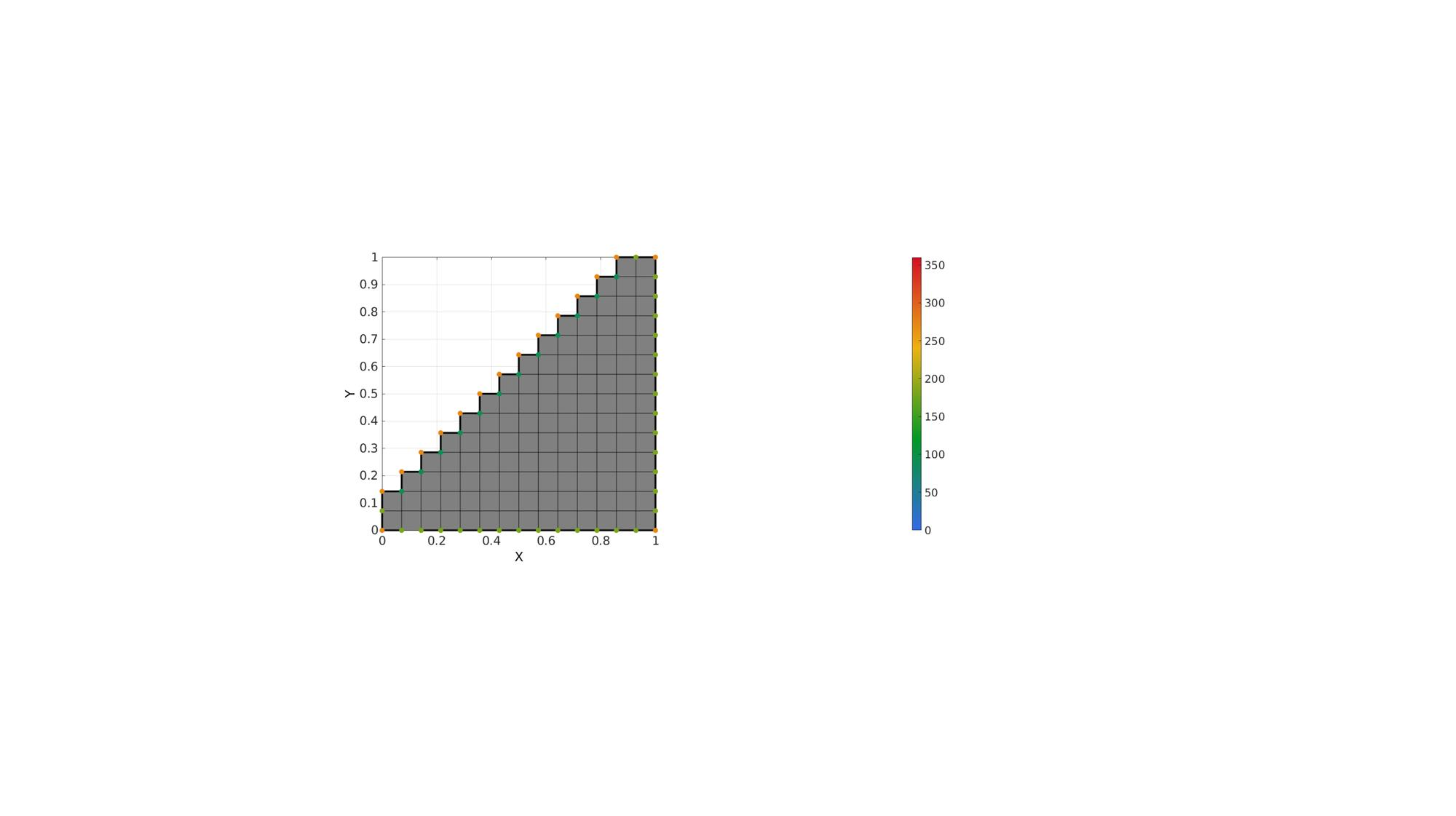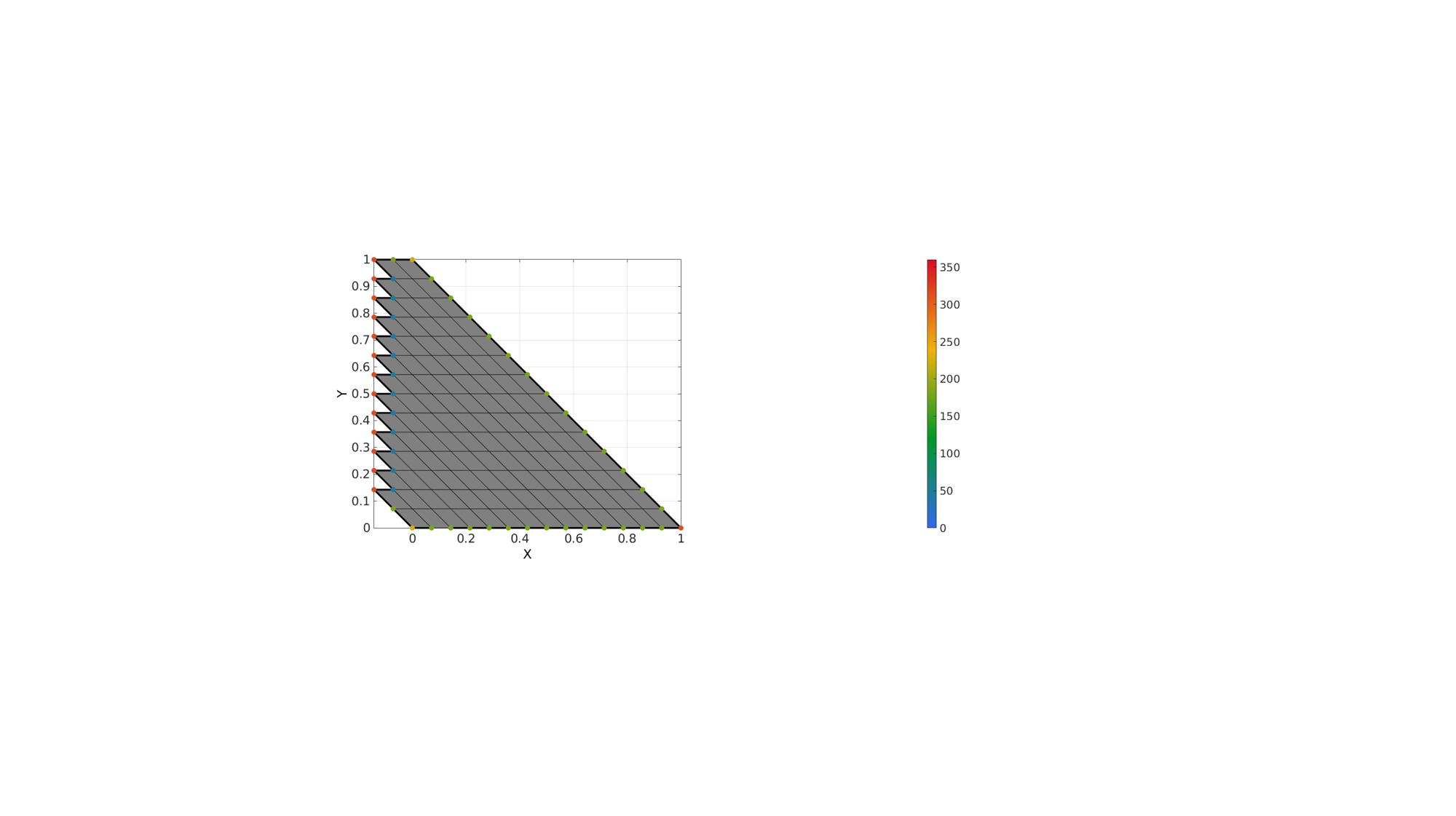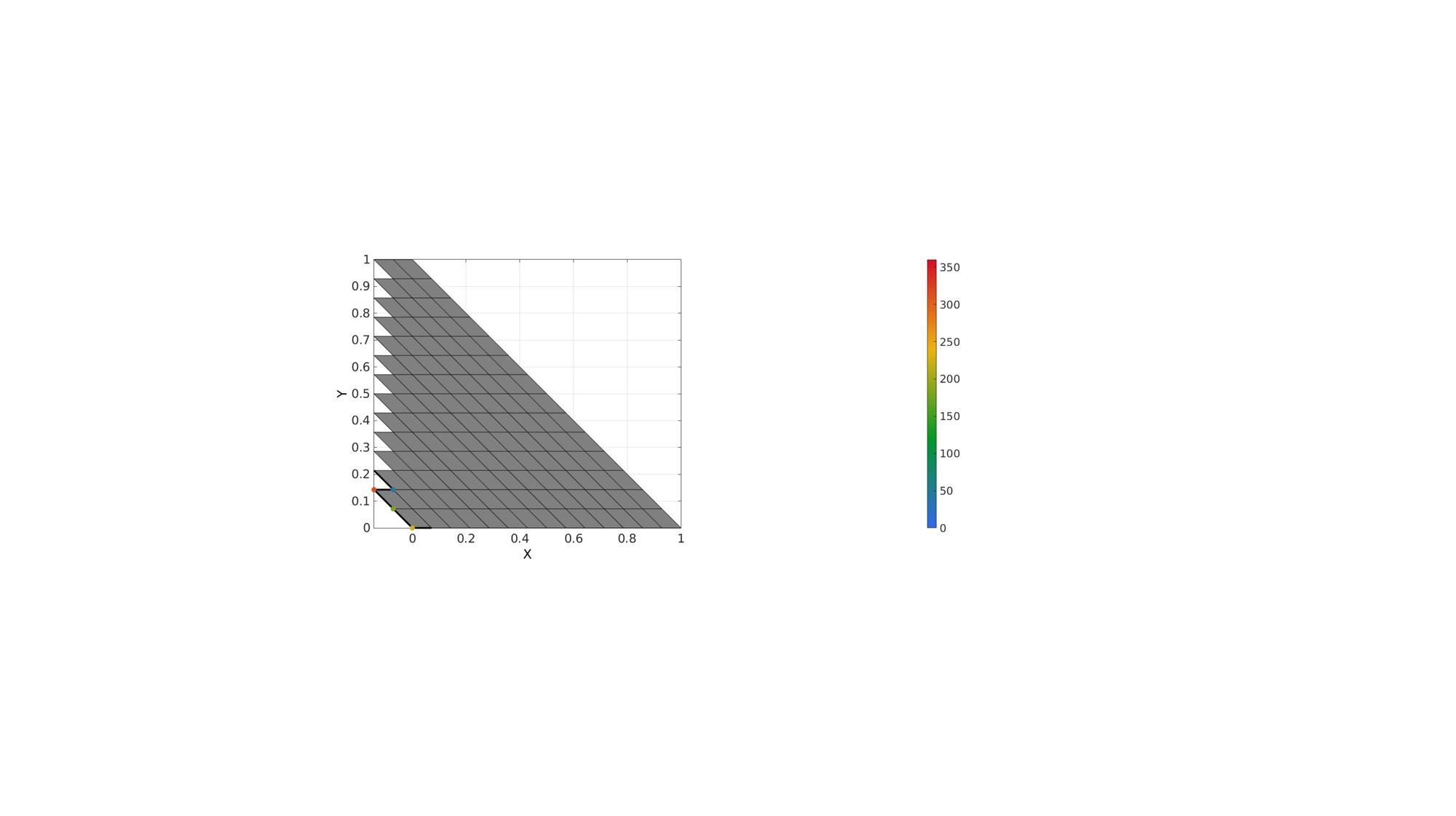# patchPathAngles

Below is a demonstration of the features of the patchPathAngles function

## Contents

```clear; close all; clc;
```

## Syntax

[indAngles]=patchPathAngles(F,V,ind,isClosedLoop);

## Description

The patchPathAngles function computes the angles between adjacent edges on a curve on a patch.

## Example 1: Get angles on a closed path defined on the the boundary of a patch

Create test data set

```w=1;
[X,Y]=ndgrid(linspace(0,w,15));
Z=ones(size(X));
C=tril(Z);
[F,V,C]=surf2patch(X,Y,Z,C);
C=vertexToFaceMeasure(F,C)>0;

logicKeep=C>0;
F=F(logicKeep,:);
C=C(logicKeep,:);
[F,V]=patchCleanUnused(F,V);
```

Get boundary curve

```Eb=patchBoundary(F,V);
indBoundaryCurve=edgeListToCurve(Eb);
indBoundaryCurve=indBoundaryCurve(1:end-1)'; %Start=End for closed curve so remove double entry
```

Calculate mesh path angles

```isClosedPath=1;
[A]=patchPathAngles(F,V,indBoundaryCurve,isClosedPath);
```
```A=180*(A./pi); % Conver to degrees

% Display unique angles in set
unique(A)
```
```ans =

90
180
270

```
```cFigure; hold on;
gpatch(F,V,'kw');
plotV(V(indBoundaryCurve,:),'k-','LineWidth',3);
scatterV(V(indBoundaryCurve,:),75,A,'filled');
colormap gjet; colorbar; caxis([0 360]);
axisGeom; view(2);
drawnow;
```## Example 2: Study angles for altered shape

```V(:,1)=V(:,1)-V(:,2);
```

Calculate mesh path angles

```isClosedPath=1;
[A]=patchPathAngles(F,V,indBoundaryCurve,isClosedPath);
```
```A=180*(A./pi); % Conver to degrees

% Display unique angles in set
unique(A)
```
```ans =

45
180
225
315

```
```cFigure; hold on;
gpatch(F,V,'kw');
plotV(V(indBoundaryCurve,:),'k-','LineWidth',3);
scatterV(V(indBoundaryCurve,:),75,A,'filled');
colormap gjet; colorbar; caxis([0 360]);
axisGeom; view(2);
drawnow;
```## Example 3: Get angles on a non-closed path defined on the the boundary of a patch

Create path segment

```indBoundaryCurve=indBoundaryCurve(1:6);
```

Calculate mesh path angles

```isClosedPath=0;
[A]=patchPathAngles(F,V,indBoundaryCurve,isClosedPath);
```
```A=180*(A./pi); % Conver to degrees

% Display unique angles in set
unique(A(~isnan(A)))
```
```ans =

45
180
225
315

```
```cFigure; hold on;
gpatch(F,V,'kw');
plotV(V(indBoundaryCurve,:),'k-','LineWidth',3);
scatterV(V(indBoundaryCurve,:),75,A,'filled');
colormap gjet; colorbar; caxis([0 360]);
axisGeom; view(2);
drawnow;
```GIBBON www.gibboncode.org

Kevin Mattheus Moerman, [email protected]

GIBBON footer text

GIBBON: The Geometry and Image-based Bioengineering add-On. A toolbox for image segmentation, image-based modeling, meshing, and finite element analysis.

Copyright (C) 2019 Kevin Mattheus Moerman

This program is free software: you can redistribute it and/or modify it under the terms of the GNU General Public License as published by the Free Software Foundation, either version 3 of the License, or (at your option) any later version.

This program is distributed in the hope that it will be useful, but WITHOUT ANY WARRANTY; without even the implied warranty of MERCHANTABILITY or FITNESS FOR A PARTICULAR PURPOSE. See the GNU General Public License for more details.

You should have received a copy of the GNU General Public License along with this program. If not, see http://www.gnu.org/licenses/.